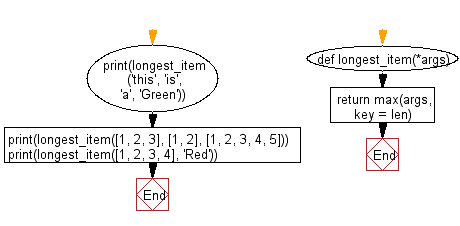﻿ Python: Find the longest one from any number of iterable objects or objects with a length property - w3resource# Python: Find the longest one from any number of iterable objects or objects with a length property

## Python List: Exercise - 245 with Solution

Write a Python program to that takes any number of iterable objects or objects with a length property and returns the longest one.

• Use max() with len() as the key to return the item with the greatest length.
• If multiple objects have the same length, the first one will be returned.

Sample Solution:

Python Code:

``````def longest_item(*args):
return max(args, key = len)
print(longest_item('this', 'is', 'a', 'Green'))
print(longest_item([1, 2, 3], [1, 2], [1, 2, 3, 4, 5]))
print(longest_item([1, 2, 3, 4], 'Red'))
```
```

Sample Output:

```Green
[1, 2, 3, 4, 5]
[1, 2, 3, 4]
```

Flowchart:## Visualize Python code execution:

The following tool visualize what the computer is doing step-by-step as it executes the said program:

Python Code Editor:

Have another way to solve this solution? Contribute your code (and comments) through Disqus.

What is the difficulty level of this exercise?

Test your Python skills with w3resource's quiz

﻿

## Python: Tips of the Day

Floor Division:

When we speak of division we normally mean (/) float division operator, this will give a precise result in float format with decimals.

For a rounded integer result there is (//) floor division operator in Python. Floor division will only give integer results that are round numbers.

```print(1000 // 300)
print(1000 / 300)```

Output:

```3
3.3333333333333335```